# Euler's Equation

Related Topics: Quaternion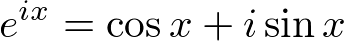Euler's equation is one of most remarkable and mysterious discoveries in Mathematics. Euler's equation (formula) shows a deep relationship between the trigonometric function and complex exponential function.

### Discovery of Euler's Equation

First, take a look the Taylor series representation of exponential function,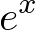and trigonometric functions, sine,and cosine,.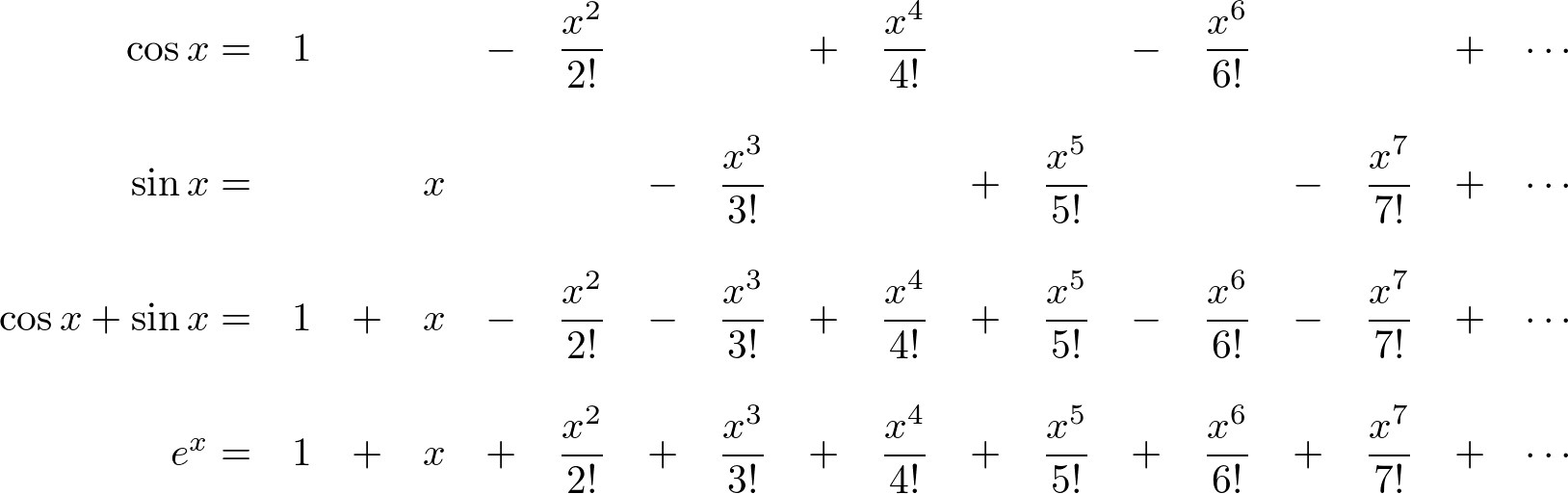Let's comparewith. Noticeis almost identical to Taylor series of; all terms in the series are exactly same except signs. As you know, the exponential function,increases exponentially as input x grows. But, what does this exponential function have to do with periodic (oscillating) functions,and?

Mathematicians had tried to figure out this weird relationship between the exponential function and the sum of 2 oscillating functions. Finally, Leonhard Euler completed this relation by bringing the imaginary number,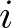into the above Taylor series;instead ofandinstead of.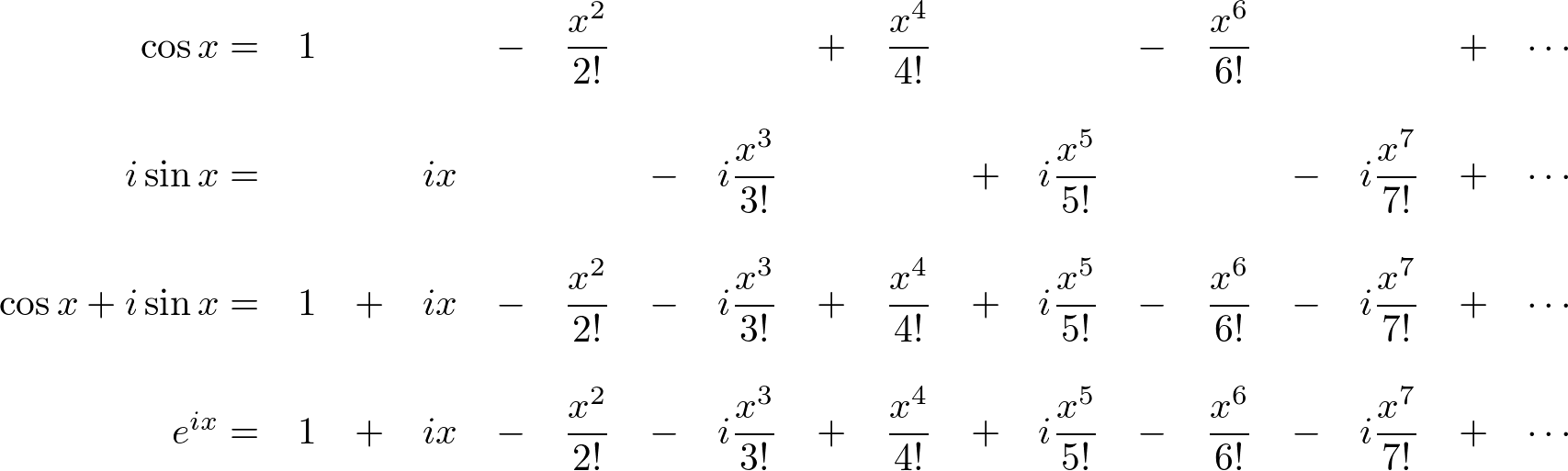Now, we find outequals to, which is known as Euler's Equation.

### Graph of Euler's Equation

We know that the exponential function,is increasing exponentially as x grows. But, what does the functionlook like?

The following images show the graph of the complex exponential function,, by plotting the Taylor series ofin the 3D complex space (x - real - imaginary axis). Surprisingly, it is a spiral spring (coil) shape, rotating around a unit circle. And, when it is projected to the real number (top view) and imaginary number axis (side view), it becomes a trigonometric function, respectively cosine and sine.Graph of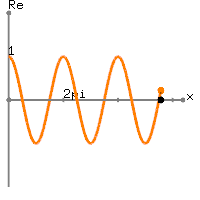Real number part of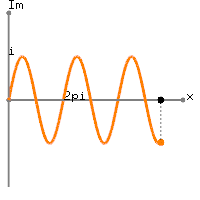Imaginary number part ofThis graphical representation of the complex exponential function,clearly shows the relation to the trigonometric function; the real number part ofis cosine and the imaginary part ofis sine function with the period ofin radians.Example of Complex Exponential Function,This is an interactive OpenGL application to draw the complex exponential function,in 3D.

euler.zip (Updated 2021-04-08)

Use the mouse cursor to rotate the view and use 'd' key to change drawing modes.

### Meaning of Euler's EquationGraph ofon the complex plane

When the graph ofis projected to the complex plane, the functionis tracing on the unit circle. It is a periodic function with the period.

It means that raising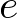to an imaginary powerproduces the complex number with the angle x in radians. This polar form ofis very convenient to represent rotating objects or periodic signals because it can represent a point in the complex plane with only single term instead of two terms,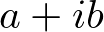. Plus, it simplifies the mathematics when used in multiplication, for example,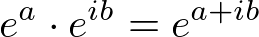.

The complex exponential forms are frequently used in electrical engineering and physics. For example, a periodic signal can be represented the sum of sine and cosine functions in Fourier analysis, and the movement of a mass attached to a string is also sinusodial. These sinusodial functions can be replaced with the complex exponential forms for simpler computation.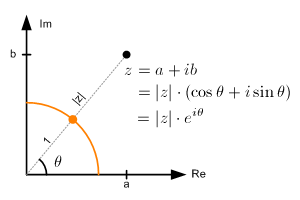Polar form of a complex number

We can extend this polar form representation for any complex number.The magnitude (norm), |z| of the complex number is a scalar value and can be rewrtten by using the laws of exponent and logarithm: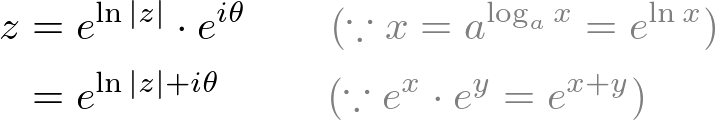### 2D Rotation with Euler's Equation

The multiplication of two complex numbers implies a rotation in 2D space.Multiplication implies rotation

Take a look at the following figure showing 2 complex numbers on the unit circle of the complex plane.
When we compare these two complex numbers, we notice that the sum of angles in the imaginary exponential form equals to the product of two complex numbers,. It tells us multiplying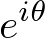by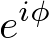performs rotatingwith angle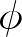.

We can extend it to any arbitary complex number. In order to rotate a complex number z = x + iy with a certain angle, we simply multiplyto the number. Then, the rotated result z' becomes.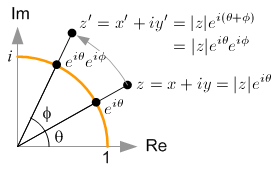2D rotation of a complex numberIf we re-write it as a matrix form by omitting, it becomes a 2x2 rotation matrix that we are familiar with.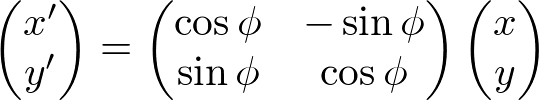It can be extended for 3D rotation by multiplying quaternion instead. Note that quaternion has a rotation axis vector u, instead of a complex number i.### Euler Identity

If we substitute the valueinto Euler's equation, then we get:This equation is called Euler Identity showing the link between 5 fundamental mathematical constants; 0, 1,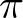,, and.

Logarithmic function is only defined for the domain x > 0. But, Euler Identity allows to define the logarithm of negative x by converting exponent to logarithm form:If we substituteto Euler's equation, then we get:Then, raise both sides to the power: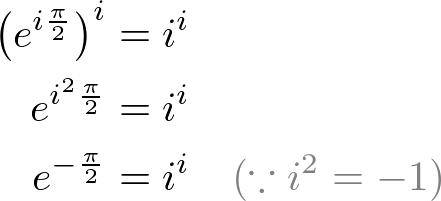The above equation tells us thatis actually a real number (not an imaginary number).

### Proof of Euler's Equation

This is a proof using calculus. Let's start with the right-hand side only and its differentiate is: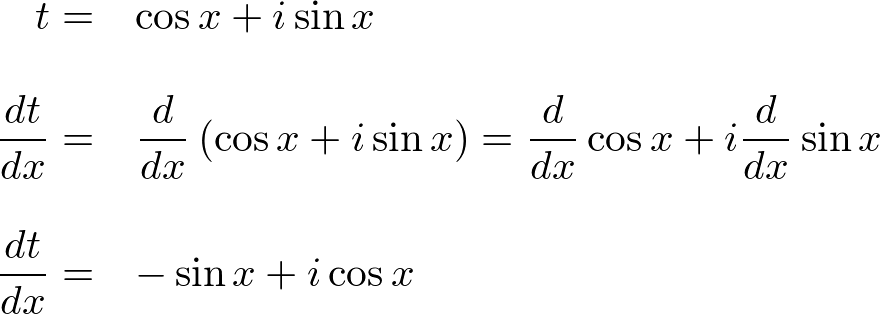Modify the right-hand side of the above equation:Move t to left-hand side, then apply integral:To find constant C, substitute x = 0:Now, we know C = 0, so the above equation is:Finally, convert this logarithm form to the exponential form: